Courses

# Process Costing - Overheads B Com Notes | EduRev

## B Com : Process Costing - Overheads B Com Notes | EduRev

The document Process Costing - Overheads B Com Notes | EduRev is a part of the B Com Course Cost Accounting.
All you need of B Com at this link: B Com

What is process costing?
A manufacturing unit that can differentiate its processes and produces a standard product will use process costing method to determine cost of production. This is done by allocating all process cost to the total units produced.
In simple words, if an unit passes through different processes and the processes are easily distinguishable then the cost of the unit will be cost of process that it goes through.
In process costing a separate account is opened for every process and on completion of the process the cost is transferred to the next process.

Illustration
A paper manufacturing unit has the following processes

• Making pulp
• Beating
• Pulp to paper
• Finishing

Let us assume  the costs incurred for producing 1000 units is

 Making  pulp Rs. 10000 Beating Rs. 20000 Pulp to paper Rs. 15000 Finishing Rs. 30000 Total Costs Rs. 75000 Total units 1000 Cost per unit Rs. 75

The above illustration is a simple presentation of process costing.  However in an actual unit there will be Work in Progress at any given period end.  So the steps for process costing will be

• To determine the total production that is: Opening stock at beginning of period + Production during the period – Rejections if any +  Equivalent units of Work in process

(To convert the number of WIP units to finished product equivalent lets understand this example : Say 500 units are in WIP and they are 50% complete then the finished product equivalent will be 500*50% =250)

• To determine the total costs, both direct and indirect costs incurred for the opening stock and current production are to be considered.
• Allocation of the costs incurred for the entire inventory is done by dividing the total costs by equivalent units of production.

As we know, there will be Work in progress during the opening and closing of a period and thus an organization needs to decide on the cost flow assumptions. For computing costs under process costing the organization can use either the FIFO or weighted average cost flow assumption.
Under the FIFO method it is assumed that the material that comes in first is transferred out first and hence the two methods (FIFO and weighted average) vary while treating opening inventory. The treatment of Work in progress in both the methods is as follows :

 Steps Weighted Average First in first out Total production Units transferred out+  Ending inventory WIP Beginning WIP+Units transferred out+ ending inventory WIP Total Costs Beginning WIP costs+ costs incurred during the period Total costs incurred during the period Cost allocation Total costs /total units Total costs /total units

The above is a simple chart differentiating the two methods.  In case of WIP there will be percentage of completion that will have to be looked into and equivalent units will have to be computed. Let us understand the same with an example,  the assumption is that the percentage of completion is the same for both material and labour.

 Opening inventory 5000, 40% complete Units started during the period 12000 Closing inventory 4000,60% complete

Under the FIFO method,  the computation of total production will be as follows :

Opening inventory – 5000*60% ( since 40% has been completed) = Rs.  3000

Completed units ( Units started – closing inventory) – 12000-4000= Rs.   8000Closing inventory – 4000*60%= Rs.  2400

Total production is 3000+8000+2400= Rs.  13400

Taking the same example let us calculate total production under the weighted average method
Completed units 12000-4000= Rs. 8000
Closing WIP 4000*60%= Rs.  2400
Total production= Rs. 10400

Similarly the costs will have to be determined and assigned to the Equivalent units of production.
In our day to day lives too there are many activities that can be segregated into defined processes. And the time involved can be assigned to each process. The cumulative time for all the processes will be the time of the entire activity.

What does process costing include?
Process costing includes the following methods of costing :

Unit Costing
This method applies to businesses that produce a single product. In this method the total cost is divided by number of units to derive per unit cost.
This is mostly used in industries dealing in- Cement manufacturing,  paper manufacturing, textiles.

Operating costing
This applies to service industry wherein the services are defined and distinguished.
This is mostly used by  Hospitals, Railways.

Operation Costing
This is similar to process costing. The only difference being that instead of process the cost for various operations is considered.
This is mostly used in industries dealing in Toy manufacturing
Therefore process costing is widely used method of costing. It can be easily applied to manufacturing as well as service industries.

Example And Solution.
Process Costing Problem 1:
The Bharat Manufacturing Company’s product passes through two distinct processes, X and Y, and then to the finished stock. It is known from the past experience that wastage occurs in the process as under:
In Process X, 5% of the units entering the process.
In Process Y, 10% of the units entering the process.
The scrap value of the wastages in process X is Rs.8 per 100 units and in process Y is Rs.10 per 100 units.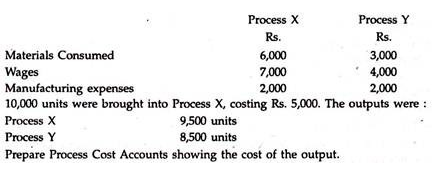Solution.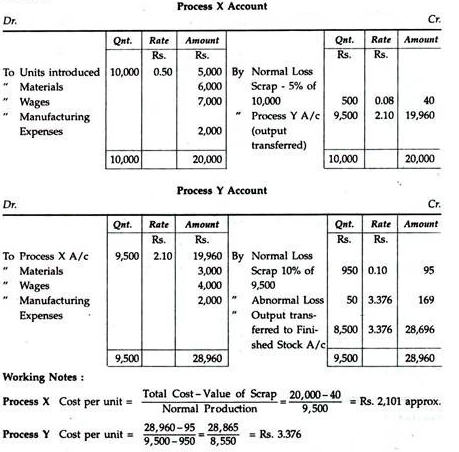Process Costing Problem 2:
ABC Ltd. is producing the product “P” required to be processed in three continuous processes. They have laid down the standards to produce 75 litres of finished product as under: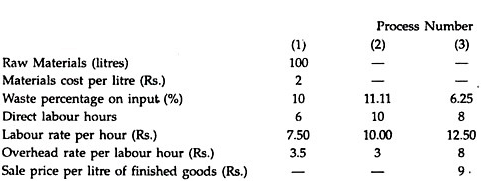You are required to prepare the statement of standard cost and standard profit rate per75 litres of finished product.
Solution.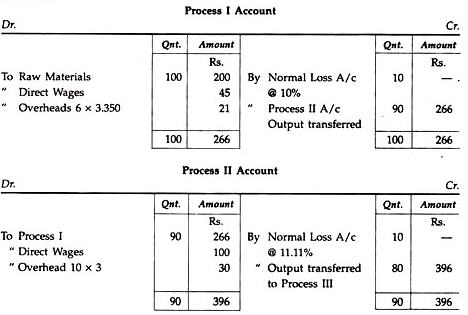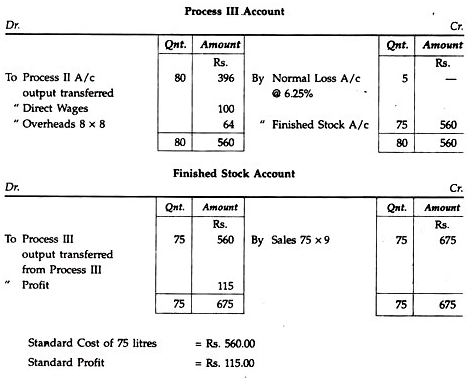Process Costing Problem 3:
The product of a manufacturing concern passes through two processes A and B and then to finished stock. It is ascertained that in each process normally 5% of the total weight is lost and 10% is scrap which from processes A and B realizes Rs.80 per ton and Rs.200 per ton, respectively:
The following are the figures relating to both the processes: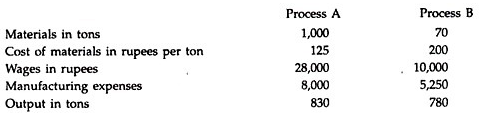Prepare Process Accounts showing cost per ton of each process. There was no stock or work-in-progress in any process.
Solution.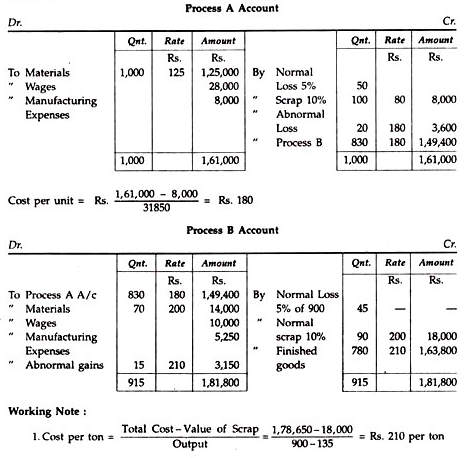Process Costing Problem 4:
A product passes through 3 distinct processes to completion. During January, 1998, 500 units were produced. The cost books show the following information: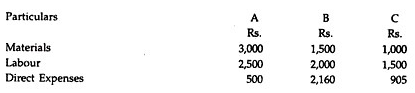The indirect expenses for the period were Rs.1,400. The by-products of process B were sold for Rs. 145. Residue of process C was sold for Rs.166. Prepare the accounts in respect of the processes showing its cost and cost of production of finished product per unit.
Solution.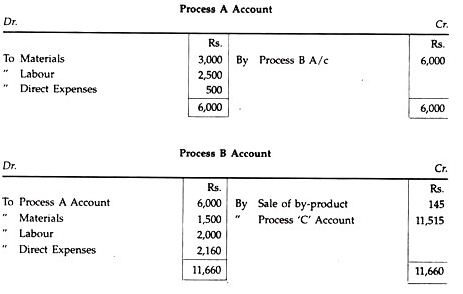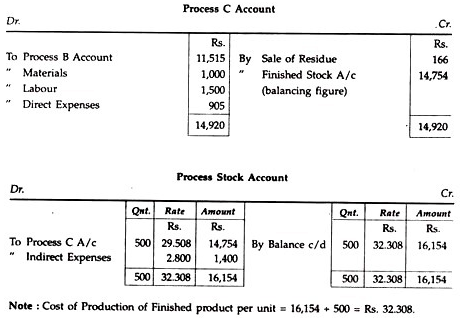Process Costing Problem 5:
The manufacture of product “Fanta” requires three distinct processes. On completion of the product is passed from Process 3 to finished stock. During the month of December 1992, the following information was obtained: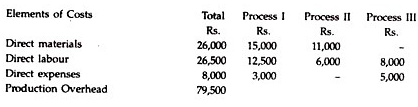Production Overhead is absorbed by processes at a percentage of direct wages. Production during the period was 1,000 kgs. There was no stock of raw materials or work-in-progress at the beginning or at the end of the month.
Solution.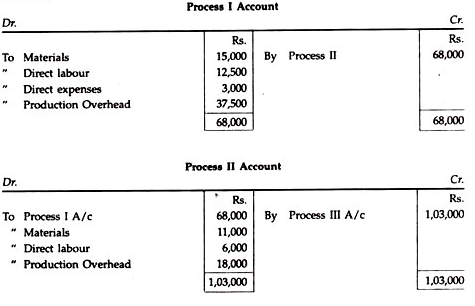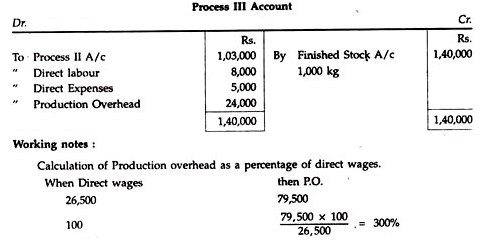Offer running on EduRev: Apply code STAYHOME200 to get INR 200 off on our premium plan EduRev Infinity!

## Cost Accounting

131 videos|139 docs

,

,

,

,

,

,

,

,

,

,

,

,

,

,

,

,

,

,

,

,

,

;